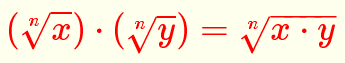Grade 10 questions on how to multiply expressions with radicals including their solutions are presented.

Product (Multiplication) of Radicals With the Same Index

Multiplication formula of radicals with equal indices is given byExamplesUse the above multiplication formula to simplify the following expressionsSolutions to the Above Problems( √x is supposed to be a real number and therefore x ? 0 which also leads |x|=x)(8√x is a real number and therefore x ? 0 which leads to |x| = x)

More
High School Math (Grades 10, 11 and 12) - Free Questions and Problems With Answers
More Middle School Math (Grades 6, 7, 8, 9) - Free Questions and Problems With Answers
More Primary Math (Grades 4 and 5) with Free Questions and Problems With Answers
Author - e-mail